Test: Sinusoidal Steady‐State Analysis - 3

# Test: Sinusoidal Steady‐State Analysis - 3

Test Description

## 10 Questions MCQ Test Network Theory (Electric Circuits) | Test: Sinusoidal Steady‐State Analysis - 3

Test: Sinusoidal Steady‐State Analysis - 3 for Electrical Engineering (EE) 2022 is part of Network Theory (Electric Circuits) preparation. The Test: Sinusoidal Steady‐State Analysis - 3 questions and answers have been prepared according to the Electrical Engineering (EE) exam syllabus.The Test: Sinusoidal Steady‐State Analysis - 3 MCQs are made for Electrical Engineering (EE) 2022 Exam. Find important definitions, questions, notes, meanings, examples, exercises, MCQs and online tests for Test: Sinusoidal Steady‐State Analysis - 3 below.
Solutions of Test: Sinusoidal Steady‐State Analysis - 3 questions in English are available as part of our Network Theory (Electric Circuits) for Electrical Engineering (EE) & Test: Sinusoidal Steady‐State Analysis - 3 solutions in Hindi for Network Theory (Electric Circuits) course. Download more important topics, notes, lectures and mock test series for Electrical Engineering (EE) Exam by signing up for free. Attempt Test: Sinusoidal Steady‐State Analysis - 3 | 10 questions in 30 minutes | Mock test for Electrical Engineering (EE) preparation | Free important questions MCQ to study Network Theory (Electric Circuits) for Electrical Engineering (EE) Exam | Download free PDF with solutions
 1 Crore+ students have signed up on EduRev. Have you?
Test: Sinusoidal Steady‐State Analysis - 3 - Question 1

### A relay coil is connected to a 210 V, 50 Hz supply. If it has resistance of 30Ω and an inductance of 0.5 H, the apparent power is

Detailed Solution for Test: Sinusoidal Steady‐State Analysis - 3 - Question 1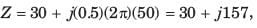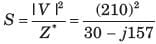Apparent power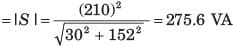Test: Sinusoidal Steady‐State Analysis - 3 - Question 2

### In the circuit shown in fig. P1.7.35 power factor is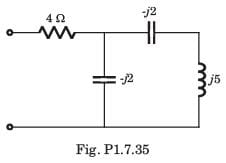Detailed Solution for Test: Sinusoidal Steady‐State Analysis - 3 - Question 2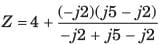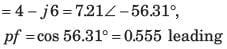Test: Sinusoidal Steady‐State Analysis - 3 - Question 3

### The power factor seen by the voltage source is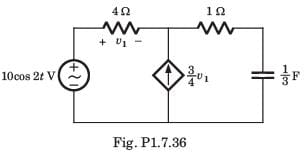Detailed Solution for Test: Sinusoidal Steady‐State Analysis - 3 - Question 3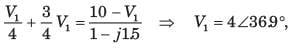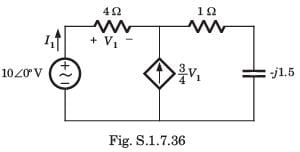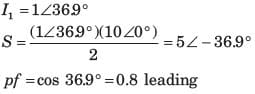Test: Sinusoidal Steady‐State Analysis - 3 - Question 4

The average power supplied by the dependent source is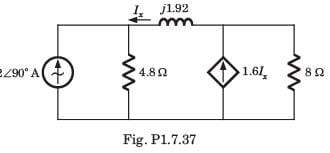Detailed Solution for Test: Sinusoidal Steady‐State Analysis - 3 - Question 4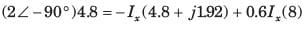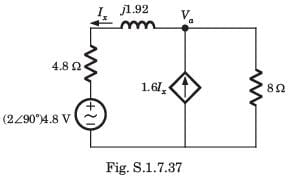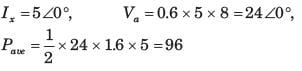Test: Sinusoidal Steady‐State Analysis - 3 - Question 5

In the circuit of fig. P1.7.38 the maximum power absorbed by ZL is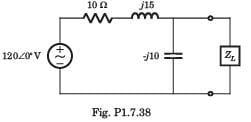Detailed Solution for Test: Sinusoidal Steady‐State Analysis - 3 - Question 5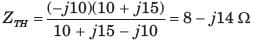Test: Sinusoidal Steady‐State Analysis - 3 - Question 6

The value of the load impedance, that would absorbs the maximum average power is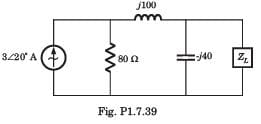Detailed Solution for Test: Sinusoidal Steady‐State Analysis - 3 - Question 6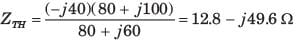Test: Sinusoidal Steady‐State Analysis - 3 - Question 7

In a balanced Y–connected three phase generator Vab = 400 Vrms
Q. If phase sequence is abc then phase voltage Va, Vb,and Vc are respectively

Detailed Solution for Test: Sinusoidal Steady‐State Analysis - 3 - Question 7

Test: Sinusoidal Steady‐State Analysis - 3 - Question 8

In a balanced Y–connected three phase generator Vab = 400 Vrms
Q. If phase sequence is acb then phase voltage are

Detailed Solution for Test: Sinusoidal Steady‐State Analysis - 3 - Question 8

For the acb sequence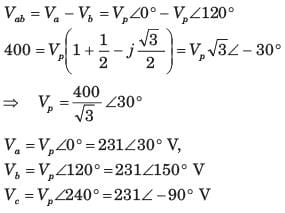Test: Sinusoidal Steady‐State Analysis - 3 - Question 9

A balanced three-phase Y-connected load has one phase voltage Vc = 277∠450V. The phase sequence is abc. The line to line voltage VAB is

Detailed Solution for Test: Sinusoidal Steady‐State Analysis - 3 - Question 9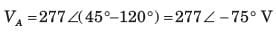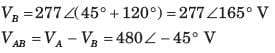Test: Sinusoidal Steady‐State Analysis - 3 - Question 10

A three-phase circuit has two parallel balanced Δ loads, one of the 6Ω resistor and one of 12 Ω resistors. The magnitude of the total line current, when the line-to-line voltage is 480 Vrms ,is

Detailed Solution for Test: Sinusoidal Steady‐State Analysis - 3 - Question 10

Z A = 6 ||12 = 4,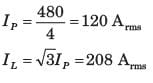## Network Theory (Electric Circuits)

23 videos|21 docs|27 tests
 Use Code STAYHOME200 and get INR 200 additional OFF Use Coupon Code
Information about Test: Sinusoidal Steady‐State Analysis - 3 Page
In this test you can find the Exam questions for Test: Sinusoidal Steady‐State Analysis - 3 solved & explained in the simplest way possible. Besides giving Questions and answers for Test: Sinusoidal Steady‐State Analysis - 3, EduRev gives you an ample number of Online tests for practice

## Network Theory (Electric Circuits)

23 videos|21 docs|27 tests